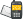It is currently 23 Mar 2019, 20:48### GMAT Club Daily Prep

#### Thank you for using the timer - this advanced tool can estimate your performance and suggest more practice questions. We have subscribed you to Daily Prep Questions via email.

Customized
for You

we will pick new questions that match your level based on your Timer History

Track

every week, we’ll send you an estimated GMAT score based on your performance

Practice
Pays

we will pick new questions that match your level based on your Timer History

#### Not interested in getting valuable practice questions and articles delivered to your email? No problem, unsubscribe here.# If x^2/4 is an integer greater than 50 and x is a positive iQuestion banks Downloads My Bookmarks Reviews Important topics
Author Message
TAGS:ModeratorJoined: 18 Apr 2015
Posts: 5877
Followers: 95

Kudos [?]: 1154  , given: 5471

If x^2/4 is an integer greater than 50 and x is a positive i [#permalink]
1
KUDOS
Expert's post00:00

Question Stats:64% (01:10) correct35% (01:11) wrongbased on 17 sessions

If $$\frac{x^2}{4}$$ is an integer greater than 50 and x is a positive integer, then what is the smallest possible value for $$x^2$$?

[Reveal] Spoiler: OA
256

_________________
InternJoined: 10 Aug 2017
Posts: 2
Followers: 0

Kudos [?]: 0 , given: 0

Re: If x^2/4 is an integer greater than 50 and x is a positive i [#permalink]
how could it possible????

Posted from my mobile deviceModeratorJoined: 18 Apr 2015
Posts: 5877
Followers: 95

Kudos [?]: 1154  , given: 5471

Re: If x^2/4 is an integer greater than 50 and x is a positive i [#permalink]
1
KUDOS
Expert's post
$$\frac{x^2}{4} > 50$$

50*4 = 200 which means that $$x^2$$ must be > 200.

The only number > 200 AND at the same time the smallest possible value is 16. $$16^2 = 256$$

Notice also that X must be a number divisible by 4.

Hope this helps.

Regards
_________________
InternJoined: 14 Jun 2018
Posts: 36
Followers: 0

Kudos [?]: 7 , given: 100

Re: If x^2/4 is an integer greater than 50 and x is a positive i [#permalink]
Carcass wrote:
$$\frac{x^2}{4} > 50$$

50*4 = 200 which means that $$x^2$$ must be > 200.

The only number > 200 AND at the same time the smallest possible value is 16. $$16^2 = 256$$

Notice also that X must be a number divisible by 4.

Hope this helps.

Regards

Thanks Carcass, it's clear. But why you started off from 16? Why if the number is extremely large? I think this could works with small numbers, but it might get knotty with complex once.
ModeratorJoined: 18 Apr 2015
Posts: 5877
Followers: 95

Kudos [?]: 1154 , given: 5471

Re: If x^2/4 is an integer greater than 50 and x is a positive i [#permalink]
Expert's post
Please read carefully my explanation on why I considered 16 as a number and above.

Regards
_________________InternJoined: 04 May 2017
Posts: 36
Followers: 0

Kudos [?]: 22  , given: 6

Re: If x^2/4 is an integer greater than 50 and x is a positive i [#permalink]
1
KUDOS
$$x^2/4>50$$ and $$x^2,x^2/4$$ is an integer <-> $$x^2>200$$ and $$x^2$$ are an integers.

We see that $$14^2=196, 15^2=225, 16^2=256$$, but $$14^2=196<250$$, $$15^2=225$$ is not divisible by 4.

So the answer is 256.
_________________

Do not pray for an easy life, pray for the strength to endure a difficult one - Bruce LeeRe: If x^2/4 is an integer greater than 50 and x is a positive i   [#permalink] 10 Jul 2018, 01:56
Display posts from previous: Sort by

# If x^2/4 is an integer greater than 50 and x is a positive iQuestion banks Downloads My Bookmarks Reviews Important topicsPowered by phpBB © phpBB Group Kindly note that the GRE® test is a registered trademark of the Educational Testing Service®, and this site has neither been reviewed nor endorsed by ETS®.